Hunting for the Optimal AutoML Library

Tech Blog Aimee Coelho

In the last few years a lot of research has been done into how to automate the process of building machine learning models. There is now a wide array of open source libraries offering AutoML. How do they relate to one another, and more importantly, how do they compare? To answer that, we’d need an independent team to test all the software packages under controlled conditions on a wide range of datasets.

Marc-André Zöller and Marco F. Huber in their paper, , have done a thorough benchmarking. We found their takeaways very interesting, and that is what we wanted to share here. Spoiler alert:

On average, all algorithms except grid search produce similarly performant models."What AutoML Means

First, it’s important to define what is included when we talk about AutoML. It’s a very broad term and can mean everything in a machine learning project from data cleaning and feature engineering to hyperparameter search and beyond to deployment to production and post-deployment monitoring for drift and redeployment of updated models.

In the context of this paper, we are looking at AutoML tools that can create a pipeline to handle data preprocessing, model selection, and hyperparameter tuning

We can break this pipeline down into the following steps: structure search and combined algorithm selection and hyperparameter search (CASH), as shown in figure 1.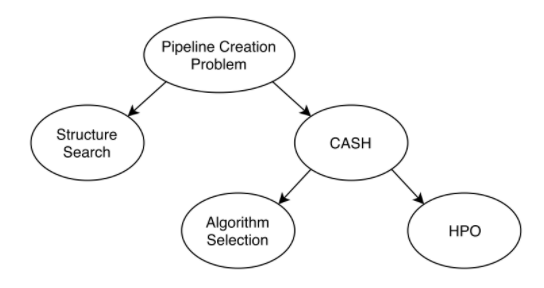Figure 1: The automatic creation of a machine learning pipeline can be broken down into a search for the structure of the pipeline and a combined algorithm selection and hyperparameter optimization. 

In the paper they divide the tools into those that perform CASH and maintain a linear pipeline — such as in figure 2 — and those capable of searching for more complex pipelines, which may include parallel branches and the recombining of data, for example see figure 3. This is the structure search in figure 1.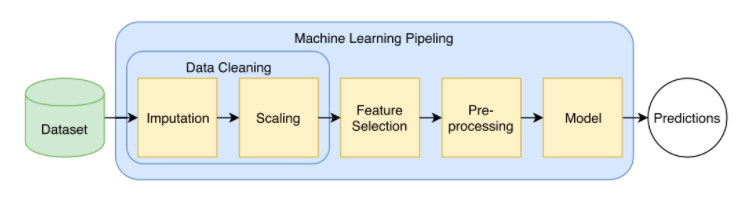Figure 2: An example of a fixed pipeline, including data preprocessing and model selection and tuning. This is the pipeline created by all the CASH tools benchmarked in the paper.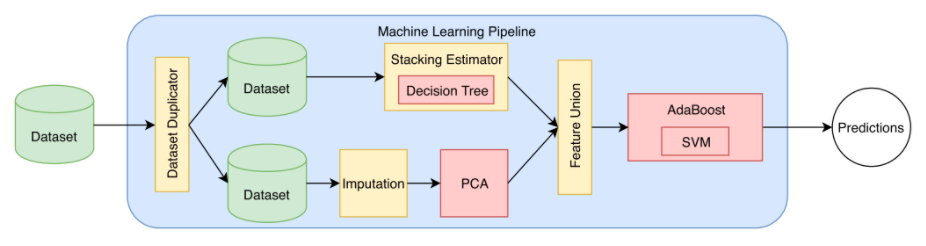Figure 3: An example of a more complex pipeline designed for a specific task. This type of pipeline is created by the AutoML platforms benchmarked in the paper. 

In their experiments they found that the CASH algorithms performed better; we will focus on these tools here.

Overview of Available Techniques

The different techniques available for conducting a hyperparameter search can be summarized as follows:

Grid Search and Random Search

First the simplest: a grid search of fixed hyperparameter values to evaluate where you simply select the best model seen.

Next is random search, where you generate random combinations of hyperparameter values based on specified distributions and ranges for each hyperparameter.

This offers the advantage of not missing the best values if they fall between two grid points, as seen in figure 4. This will get arbitrarily close to optimum if run indefinitely, unlike grid search.

For both grid and random search, the authors chose the scikit-learn implementations.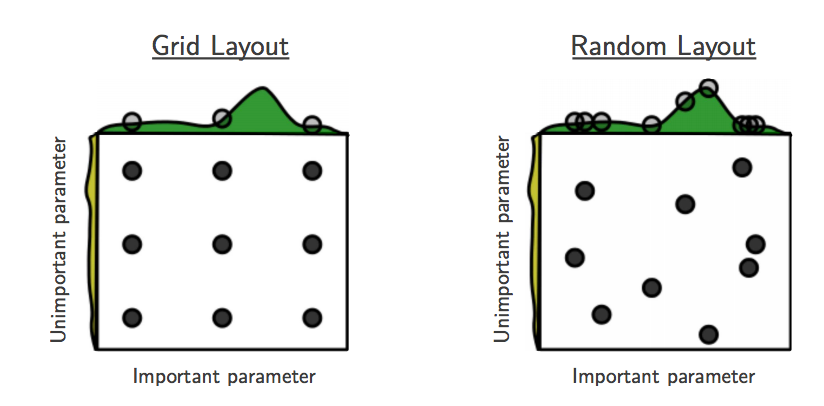Figure 4: Using a fixed grid of points for the hyperparameter search can miss the best values of some hyperparameters, this effect becomes more pronounced in higher dimensional searches. 

Sequential Model-Based Optimization Search

Then we have sequential model based optimization (). The goal here is to learn from the evaluations as they are completed, similar to how a data scientist would construct a hyperparameter search. These techniques learn a surrogate model after each evaluation, then they use an acquisition function that can query the surrogate model to decide which point to evaluate next. Querying the surrogate model is much faster than training a model to get the true performance value.

Model-based optimization techniques aim to learn the most promising region of the search space and explore this more thoroughly in order to find the best model.

Example. Consider a simple two-dimensional quadratic function with a minima at X_0 = 50 and X_1 = -50. When using Bayesian optimization to optimize this function, after first evaluating 10 random configurations to learn a surrogate model on, the later evaluations begin to cluster closer and closer toward the minimum. In the left of figure 5 this objective function is plotted, and on the right — the sequence of points chosen by the Bayesian optimization algorithm with Gaussian processes in they explore more densely the region around the minimum as the number of iterations increases from purple to red.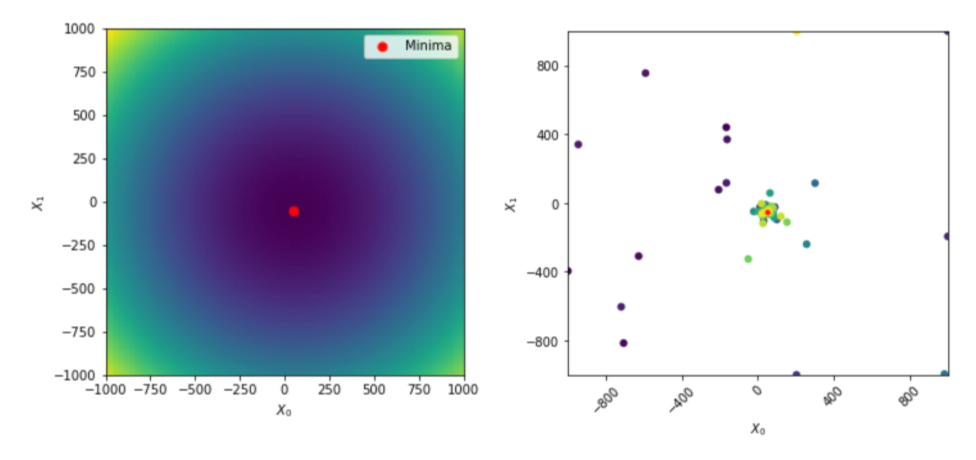Figure 5: On the left, a plot of the quadratic function we are trying to optimize. On the right, a plot showing the successive points chosen (from purple to red) to evaluate while running scikit-optimize to normalize this function using Bayesian optimization with Gaussian processes. As the number of iterations increases, the algorithm explores more closely the region around the minimum.

Different choices are available for the surrogate model and include Gaussian Processes, Random Forests and . The authors selected to test  for SMBO with Gaussian Processes,  for SMBO with Random Forests and  for SMBO with Tree Parzen Estimators.

Other Types of Search

It is also possible to combine multi-armed bandit learning with Bayesian optimization, the choice of categorical parameters is handled by the bandit and breaks the space into subspaces called hyper-partitions each of which contains only continuous parameters which are optimized by Bayesian optimization. The Bayesian Tuning Bandits () implementation was selected for this benchmark.

Another approach is to use evolutionary algorithms; there are a range of different population-based algorithms in use, such as Particle Swarm Optimization. The Particle Swarm Optimization from  was used in this benchmark.

Performance Enhancements

In addition to these main algorithms, there have been many proposals for how to enhance these techniques — they are mostly geared toward reducing the time required to find the optimum model.

Low Fidelity

One popular approach is to use lower fidelity evaluations. This could be training on samples of the data or a smaller number of iterations of training for a model — since this is a much faster way to get an idea of the performance of each configuration, more configurations can be tested early on and only the most promising configurations trained fully.

One good example of this is .  is a tool using a combination of hyperband and Bayesian optimization and is included in this benchmark.

Meta Learning

A clear way to speed up model-based optimization would be if you already had an idea of the most promising region of the search space to explore. Then, you could narrow down the search from the beginning without starting from a random search. One way to do this would be to study which are generally the most and what are commonly good values for them for a given algorithm on a wide range of datasets.

Another way is to use results from previous hyperparameter searches you may have done on other datasets. If you can identify the most similar datasets, you can use the best hyperparameters from that task to start your search — this is known as warm-starting the search.

One example of where this is implemented is in , and more details about how you can assess dataset similarity can be found in their .

Benchmarking Experiments

The data used for this benchmark was a combination of the curated benchmarking suites OpenML100 , OpenML-CC18 , and AutoML Benchmark , in total 137 classification datasets.

All libraries were run for 325 iterations, as this was determined to be long enough for all algorithms to converge, and any single model training was limited to 10 minutes.

The libraries had 13 different algorithms with a total of 58 hyperparameters to optimize. The performance of each configuration is evaluated using four-fold cross validation, and the experiments are repeated 10 times with different random seeds.

Results

In order to compare the accuracies across datasets with different difficulty levels, two baseline models are used. One is a , and the other a simple untuned random forest. The results from these baselines are then used to normalize the results so that the performance of the dummy classifier becomes zero and the untuned random forest one. Therefore, any library performing better than one means it performs better than an untuned random forest.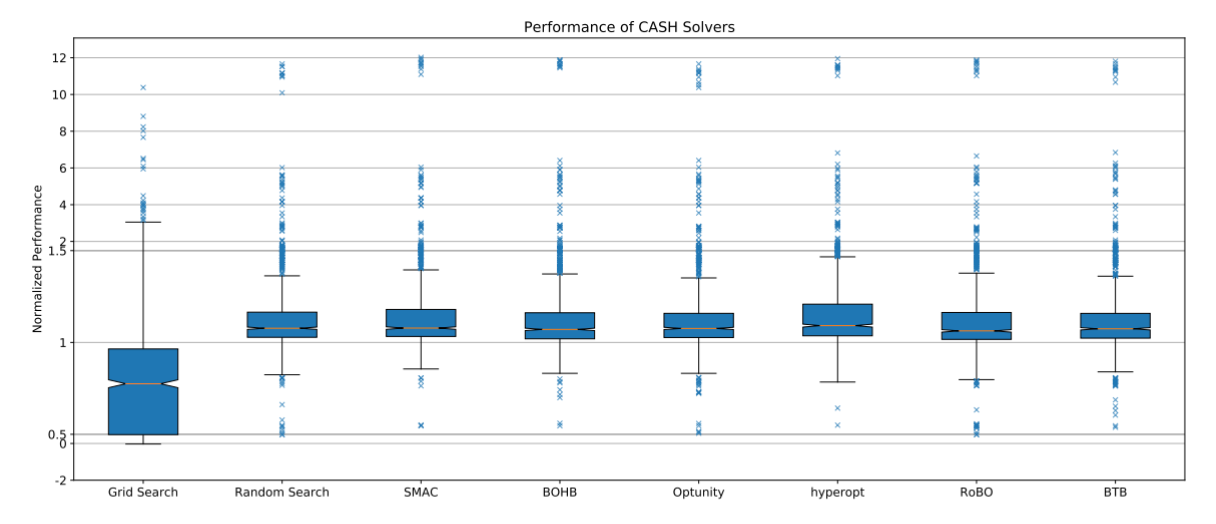Figure 6: The normalized performance of all CASH solvers tested from the paper , the regions between 0.5 and 1.5 are stretched out for increased readability.

Surprisingly they found that all tools performed similarly except for grid search!

After ten iterations all techniques except grid search were able to outperform the random forest baseline, and the final performances after all 325 iterations are shown in figure 6.

For those of you who are curious, the way that the grid for the grid search is defined is also automatic and the default parameters for random forest do not appear in the grid which is how it performs worse than an untuned random forest, the full grid is included in the appendix of the paper.

A Surprisingly High Variance in the Experiments

The authors also highlighted that some datasets showed a rather large variance when changing just the random seed. This is something we also observed in our own benchmarking experiments at , and it demonstrates the importance of repeating the experiments.

You can see this phenomenon in this clear visualization in figure 7 where the raw and averaged accuracies are plotted together for the datasets with the highest variances. For the dataset with the highest variance, diabetes, the results from random search vary by more than 20% !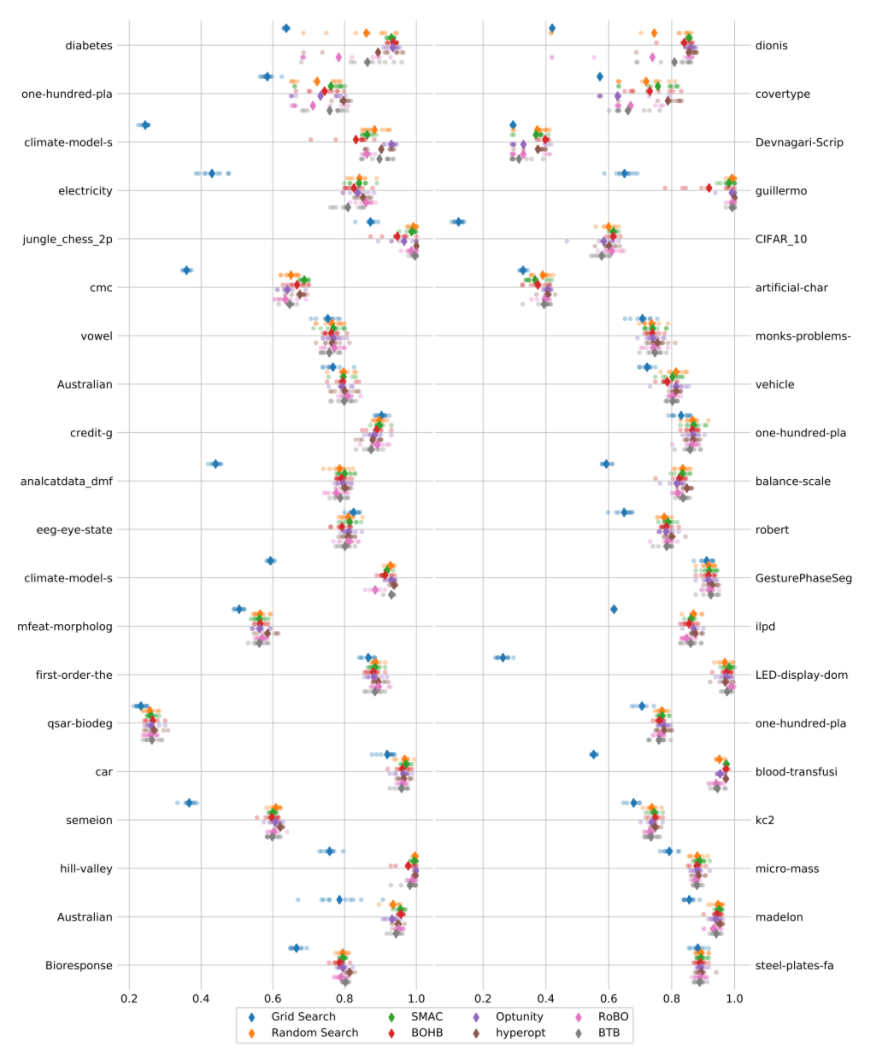Figure 7: The raw accuracies for each run with a different random seed and the average for the 40 datasets with the highest variance between seeds. 

Takeaways from the authors

The authors concluded that

On average, all algorithms except grid search produce similarly performant models."

They also concluded that on most datasets, the absolute differences in accuracy are less than 1%, and that therefore a ranking of these algorithms based purely on performance is not reasonable. For future rankings of algorithms, other criteria should be considered (for example model overhead or scalability).

Beyond Accuracy: Rate of Convergence as Selection Criterion

While ultimately the goal is to get the best possible model, in practice there are additional limitations on computing resources and time, especially when we are working with much larger data in industry where training hundreds of models might be impractical.

In this scenario, an important consideration is how quickly an algorithm can get close to the optimum model. When the resources are available to train hundreds of models, criteria such as the overhead of the model are important, but if you can only afford to train for example 20 models, then the rate of convergence becomes a very important criteria.

To illustrate this better, consider the following plot in figure 8.

On the , four search algorithms were used to optimise four hyperparameters of just a single algorithm, random forest. The best loss at each iteration is plotted. The first vertical line is placed at 20 iterations.

By this point, all model-based optimization algorithms are no longer performing random search but choosing the points to evaluate based on their own models. Between here and 50 iterations, the second vertical line, we see there is a greater difference between the performance of the models found by these techniques than after the full 325 iterations once all algorithms have reached convergence.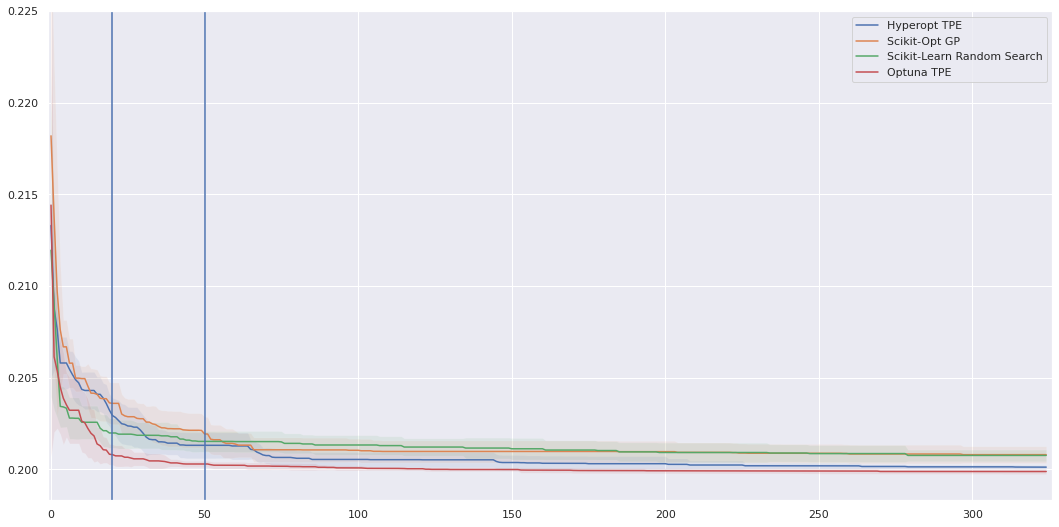Figure 8: The loss per iteration obtained using four different search algorithms to tune four hyperparameters of a simple random forest, the difference between the best loss of the models found by each search algorithm is more pronounced with fewer iterations.

Thus if you could only afford to train 20 models, you would have a better model if you chose to use a Tree Parzen Estimator-based algorithm, such as the one implemented in .

References

1. Zöller, M.-A. & Huber, M. F. Benchmark and Survey of Automated Machine Learning Frameworks. (2019).

2. Bergstra, J. & Bengio, Y. Random search for hyper-parameter optimization. J. Mach. Learn. Res. 13, 281–305 (2012).

3. Casalicchio, G., Hutter, F., Mantovani, R. G. & Vanschoren, J. OpenML Benchmarking Suites and the OpenML100. 1–6

4. Casalicchio, G., Hutter, F. & Mantovani, R. G. OpenML Benchmarking Suites. 1–6

5. Gijsbers, P. et al. An Open Source AutoML Benchmark. 1–8 (2019).